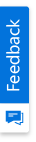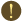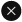We use cookies to give you the best experience on our website. If you continue to browse, then you agree to our privacy policy and cookie policy.Unfortunately, activation email could not send to your email. Please try again.# How to create dependent dropdownlist using XlsIO?

Platform: WinForms |
Control: XlsIO

XlsIO allows to create dependent dropdownlist in data validation using INDIRECT formula in data validation list source. The following steps illustrates with code snippets on how to achieve the requirement.

1. Create named ranges for the range of cells. Here we have created the 4 Named Ranges and the following table describes the named ranges and it’s refer to ranges. Add meaningful values in these ranges.

 Named Ranges Range Food A2:A4 Pizza B2:B5 PanCakes C2:C3 Chinese D2:D41. Choose a cell to create the dropdownlist and set the data validation. Here, we have selected the B8 Cell and applied data validation with list values which refers to a NamedRange (Food).
2. Select a next cell to apply data validation which depends on the previous data validation list. Here, we have selected the cell “B10” and applied data validation with list type and assigned the list source as “=INDIRECT(\$B1)”. (INDIRECT function is used to return the reference, specified by the string from the drop down list).

C#

```            //Step 1 : Instantiate the spreadsheet creation engine.
ExcelEngine excelEngine = new ExcelEngine();
//Step 2 : Instantiate the excel application object.
IApplication application = excelEngine.Excel;

//Create a workbook.
IWorkbook workbook = application.Workbooks.Create(1);
IWorksheet sheet1 = workbook.Worksheets;

//Create named ranges
IName name1 = workbook.Names.Add("Chinese");
name1.RefersToRange = sheet1["D2:D4"];
name1.Value = "Sheet1!\$D\$2:\$D\$4";

IName name2 = workbook.Names.Add("Food");
name2.RefersToRange = sheet1["A2:A4"];
name2.Value = "Sheet1!\$A\$2:\$A\$4";

IName name3 = workbook.Names.Add("PanCakes");
name3.RefersToRange = sheet1["C2:C3"];
name3.Value = "Sheet1!\$C\$2:\$C\$3";

IName name4 = workbook.Names.Add("Pizza");
name4.RefersToRange = sheet1["B2:B5"];
name4.Value = "Sheet1!\$B\$2:\$B\$5";

//Set values
IRange range1 = sheet1["A1"];
range1.Text = "FOOD";

IFont font1 = range1.CellStyle.Font;
font1.FontName = "Tahoma";
font1.Bold = true;
font1.Size = 10;

IRange range2 = sheet1["B1"];
range2.Text = "FOOD_PIZZA";

IFont font2 = range2.CellStyle.Font;
font2.FontName = "Tahoma";
font2.Bold = true;
font2.Size = 10;

IRange range3 = sheet1["C1"];
range3.Text = "FOOD_PANCAKES";

IFont font3 = range3.CellStyle.Font;
font3.FontName = "Tahoma";
font3.Bold = true;
font3.Size = 10;

IRange range4 = sheet1["D1"];
range4.Text = "FOOD_CHINESE";

IFont font4 = range4.CellStyle.Font;
font4.FontName = "Tahoma";
font4.Bold = true;
font4.Size = 10;

IRange range5 = sheet1["A2"];
range5.Text = "Pizza";

IRange range6 = sheet1["B2"];
range6.Text = "Mediterranean";

IRange range7 = sheet1["C2"];
range7.Text = "Bacon";

IRange range8 = sheet1["D2"];
range8.Text = "Almond Chiken";

IRange range9 = sheet1["A3"];
range9.Text = "PanCakes";

IRange range10 = sheet1["B3"];
range10.Text = "Pepperoni";

IRange range11 = sheet1["C3"];
range11.Text = "Cheese";

IRange range12 = sheet1["D3"];
range12.Text = "Chop Suey";

IRange range13 = sheet1["A4"];
range13.Text = "Chinese";

IRange range14 = sheet1["B4"];
range14.Text = "California";

IRange range15 = sheet1["D4"];
range15.Text = "Crab rangoon";

IRange range16 = sheet1["B5"];
range16.Text = "NewYorker";

IRange range17 = sheet1["A8"];
range17.Text = "Favourite Food";

IFont font5 = range17.CellStyle.Font;
font5.FontName = "Tahoma";
font5.Bold = true;
font5.Size = 10;

IRange range18 = sheet1["B8"];            range18.Text = "Pizza";
//Apply DataValidation
IDataValidation dataValidation1 = range18["B8"].DataValidation;
dataValidation1.AllowType = ExcelDataType.User;
dataValidation1.ErrorStyle = ExcelErrorStyle.Stop;
dataValidation1.FirstDateTime = new DateTime(1, 1, 1, 0, 0, 0);
dataValidation1.FirstFormula = "Food";
dataValidation1.IsEmptyCellAllowed = true;
dataValidation1.IsPromptBoxVisible = false;
dataValidation1.SecondDateTime = new DateTime(1899, 12, 30, 0, 0, 0); dataValidation1.SecondFormula = "0";

IDataValidation dataValidation2 = range20["B10"].DataValidation;
dataValidation2.AllowType = ExcelDataType.User;
dataValidation2.ErrorStyle = ExcelErrorStyle.Stop;
dataValidation2.FirstDateTime = new DateTime(1, 1, 1, 0, 0, 0);
dataValidation2.FirstFormula = "INDIRECT(\$B8)";
dataValidation2.IsEmptyCellAllowed = true;
dataValidation2.IsPromptBoxVisible = false;
dataValidation2.SecondDateTime = new DateTime(1899, 12, 30, 0, 0, 0);            dataValidation2.SecondFormula = "0";
```

VB

``` 'Step 1 : Instantiate the spreadsheet creation engine.
Dim excelEngine As ExcelEngine = New ExcelEngine()
'Step 2 : Instantiate the excel application object.
Dim application As IApplication = excelEngine.Excel

'Create a workbook.
Dim workbook As IWorkbook = application.Workbooks.Create(1)
Dim sheet1 As IWorksheet = workbook.Worksheets(0)

'Create named ranges
Dim name1 As IName = workbook.Names.Add("Chinese")
name1.RefersToRange = sheet1("D2:D4")
name1.Value = "Sheet1!\$D\$2:\$D\$4"

Dim name2 As IName = workbook.Names.Add("Food")
name2.RefersToRange = sheet1("A2:A4")
name2.Value = "Sheet1!\$A\$2:\$A\$4"

Dim name3 As IName = workbook.Names.Add("PanCakes")
name3.RefersToRange = sheet1("C2:C3")
name3.Value = "Sheet1!\$C\$2:\$C\$3"

Dim name4 As IName = workbook.Names.Add("Pizza")
name4.RefersToRange = sheet1("B2:B5")
name4.Value = "Sheet1!\$B\$2:\$B\$5"

'Set values
Dim range1 As IRange = sheet1("A1")
range1.Text = "FOOD"

Dim font1 As IFont = range1.CellStyle.Font
font1.FontName = "Tahoma"
font1.Bold = True
font1.Size = 10

Dim range2 As IRange = sheet1("B1")
range2.Text = "FOOD_PIZZA"

Dim font2 As IFont = range2.CellStyle.Font
font2.FontName = "Tahoma"
font2.Bold = True
font2.Size = 10

Dim range3 As IRange = sheet1("C1")
range3.Text = "FOOD_PANCAKES"

Dim font3 As IFont = range3.CellStyle.Font
font3.FontName = "Tahoma"
font3.Bold = True
font3.Size = 10

Dim range4 As IRange = sheet1("D1")
range4.Text = "FOOD_CHINESE"

Dim font4 As IFont = range4.CellStyle.Font
font4.FontName = "Tahoma"
font4.Bold = True
font4.Size = 10

Dim range5 As IRange = sheet1("A2")
range5.Text = "Pizza"

Dim range6 As IRange = sheet1("B2")
range6.Text = "Mediterranean"

Dim range7 As IRange = sheet1("C2")
range7.Text = "Bacon"

Dim range8 As IRange = sheet1("D2")
range8.Text = "Almond Chiken"

Dim range9 As IRange = sheet1("A3")
range9.Text = "PanCakes"

Dim range10 As IRange = sheet1("B3")
range10.Text = "Pepperoni"

Dim range11 As IRange = sheet1("C3")
range11.Text = "Cheese"

Dim range12 As IRange = sheet1("D3")
range12.Text = "Chop Suey"

Dim range13 As IRange = sheet1("A4")
range13.Text = "Chinese"

Dim range14 As IRange = sheet1("B4")
range14.Text = "California"

Dim range15 As IRange = sheet1("D4")
range15.Text = "Crab rangoon"

Dim range16 As IRange = sheet1("B5")
range16.Text = "NewYorker"

Dim range17 As IRange = sheet1("A8")
range17.Text = "Favourite Food"

Dim font5 As IFont = range17.CellStyle.Font
font5.FontName = "Tahoma"
font5.Bold = True
font5.Size = 10

Dim range18 As IRange = sheet1("B8")
range18.Text = "Pizza"
'Apply DataValidation
Dim dataValidation1 As IDataValidation = range18("B8").DataValidation
dataValidation1.AllowType = ExcelDataType.User
dataValidation1.ErrorStyle = ExcelErrorStyle.Stop
dataValidation1.FirstDateTime = New DateTime(1, 1, 1, 0, 0, 0)
dataValidation1.FirstFormula = "Food"
dataValidation1.IsEmptyCellAllowed = True
dataValidation1.IsPromptBoxVisible = False
dataValidation1.SecondDateTime = New DateTime(1899, 12, 30, 0, 0, 0)
dataValidation1.SecondFormula = "0"

Dim dataValidation2 As IDataValidation = range20("B10").DataValidation
dataValidation2.AllowType = ExcelDataType.User
dataValidation2.ErrorStyle = ExcelErrorStyle.Stop
dataValidation2.FirstDateTime = New DateTime(1, 1, 1, 0, 0, 0)
dataValidation2.FirstFormula = "INDIRECT(\$B8)"
dataValidation2.IsEmptyCellAllowed = True
dataValidation2.IsPromptBoxVisible = False
dataValidation2.SecondDateTime = New DateTime(1899, 12, 30, 0, 0, 0)
dataValidation2.SecondFormula = "0"
```

You can find the sample here.

2X faster development

The ultimate WinForms UI toolkit to boost your development speed.You are using an outdated version of Internet Explorer that may not display all features of this and other websites. Upgrade to Internet Explorer 8 or newer for a better experience.